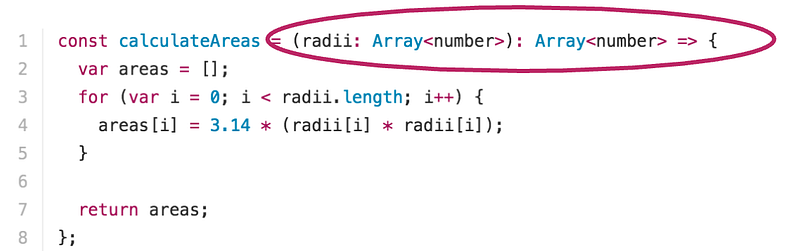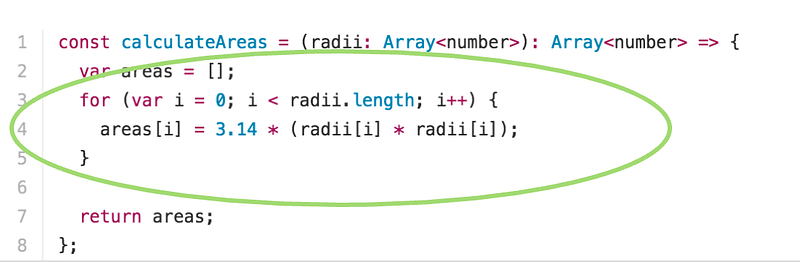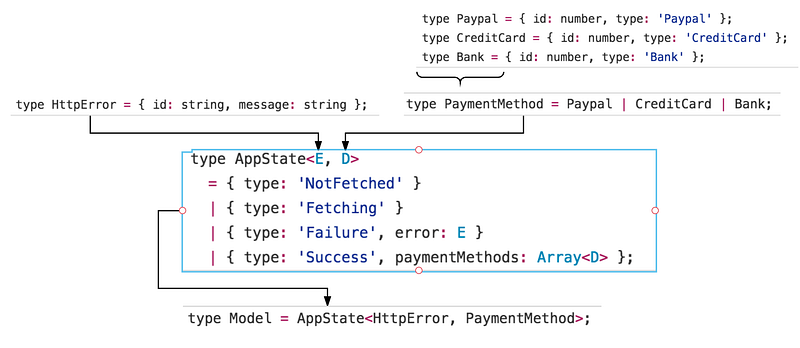# 使用静态类型的优势

## 优势一：你可以尽早发现bug和错误

``````const calculateArea = (radius) => 3.14 * radius * radius;

var area = calculateArea(3);
// 28.26
``````

``````var area = calculateArea('im evil');
// NaN
``````

``````const calculateArea = (radius: number): number => 3.14 * radius * radius;
``````

``````calculateArea('Im evil');
^^^^^^^^^^^^^^^^^^^^^^^^^ function call
calculateArea('Im evil');
^^^^^^^^^ string. This type is incompatible with

^^^^^^ number
``````

## 优势二：起到在线文档的功能

``````function calculatePayoutDate(quote, amount, paymentMethod) {
let payoutDate;

return payoutDate;
}
``````

quote参数是一个数值型么？还是一个boolean型？paymentMethod是一个对象么？还是仅仅就是一个代表着支付方式的字符串？这个方法会返回一个表示日期的字符串么？还是一个`Date`对象？

``````function calculatePayoutDate(
quote: boolean,
amount: number,
paymentMethod: string): Date {
let payoutDate;

return payoutDate;
}
``````

``````/*
@function Determines the payout date for a purchase
@param {boolean} quote - Is this for a price quote?
@param {boolean} amount - Purchase amount
@param {string} paymentMethod - Type of payment method used for this purchase
*/
function calculatePayoutDate(quote, amount, paymentMethod) {
let payoutDate;
/* .... Business logic .... */

return payoutDate;
};
``````

## 优势三：减少了复杂的错误处理

``````const calculateAreas = (radii) => {
var areas = [];
for (let i = 0; i < radii.length; i++) {
}

return areas;
};
``````

``````const calculateAreas = (radii) => {
// Handle undefined or null input
throw new Error("Argument is missing");
}

// Handle non-array inputs
throw new Error("Argument must be an array");
}

var areas = [];

for (var i = 0; i < radii.length; i++) {
if (typeof radii[i] !== "number") {
throw new Error("Array must contain valid numbers only");
} else {
}
}

return areas;
};
``````

``````const calculateAreas = (radii: Array<number>): Array<number> => {
var areas = [];
for (var i = 0; i < radii.length; i++) {
}

return areas;
};
``````

## 优势五：将数据和行为分离

``````const calculateAreas = (radii: Array<number>): Array<number> => {
var areas = [];
for (var i = 0; i < radii.length; i++) {
}

return areas;
};
``````imageimage

## 优势六：帮助我们消除了一整类bug

``````var appState = {
isFetching: false,
messages: [],
};
``````

``````import Message from './Message';

const MyComponent = ({ messages }) => {
return (
<div>
<h1> You have { messages.length } unread messages </h1>
{ messages.map(message => <Message message={ message } /> )}
</div>
);
};
``````

``````TypeError: Cannot read property ‘length’ of undefined
``````

……然后我们的程序就崩溃了。你就这么失去了你的客户。僵……

``````type AppState = {
isFetching: boolean,
messages: ?Array<string>
};

var appState: AppState = {
isFetching: false,
messages: null,
};
``````

``````import Message from './Message';

const MyComponent = ({ messages }) => {
return (
<div>
<h1> You have { messages.length } unread messages </h1>
{ messages.map(message => <Message message={ message } /> )}
</div>
);
};
``````

Flow会帮我们捕获并将错误告知我们：

``````<h1> You have {messages.length} unread  messages </h1>
^^^^^^ property `length`. Property cannot be accessed on possibly null value
<h1> You have {messages.length} unread messages </h1>
^^^^^^^^ null

<h1> You have {messages.length} unread  messages </h1>
^^^^^^ property `length`. Property cannot be accessed on possibly undefined value

<h1> You have {messages.length} unread messages </h1>
^^^^^^^^ undefined

{ messages.map(message => <Message message={ message } /> )}
^^^^^^^^^^^^^^^^^^^^^^^^^^^^^^^^^^^^^^^^^^^^^^ call of method `map`. Method cannot be called on possibly null value

{ messages.map(message => <Message message={ message } /> )}
^^^^^^^^ null

{ messages.map(message => <Message message={ message } /> )}
^^^^^^^^^^^^^^^^^^^^^^^^^^^^^^^^^^^^^^^^^^^^^^ call of method `map`. Method cannot be called on possibly undefined value

{ messages.map(message => <Message message={ message } /> )}
^^^^^^^^ undefined
``````

Wow！

``````const MyComponent = ({ messages, isFetching }: AppState) => {
if (isFetching) {
} else if (messages === null || messages === undefined) {
return <div> Failed to load messages. Try again. </div>
} else {
return (
<div>
<h1> You have { messages.length } unread messages </h1>
{ messages.map(message => <Message message={ message } /> )}
</div>
);
}
};
``````

## 优势七：减少单元测试的数量

``````const calculateAreas = (radii) => {
// Handle undefined or null input
throw new Error("Argument is missing");
}

// Handle non-array inputs
throw new Error("Argument must be an array");
}

var areas = [];

for (var i = 0; i < radii.length; i++) {
if (typeof radii[i] !== "number") {
throw new Error("Array must contain valid numbers only");
} else {
}
}

return areas;
};
``````

``````it('should not work - case 1', () => {
expect(() => calculateAreas([null, 1.2])).to.throw(Error);
});

it('should not work - case 2', () => {
expect(() => calculateAreas(undefined).to.throw(Error);
});

it('should not work - case 2', () => {
expect(() => calculateAreas('hello')).to.throw(Error);
});
``````

``````const calculateAreas = (radii: Array<number>): Array<number> => {
var areas = [];
for (var i = 0; i < radii.length; i++) {
}

return areas;
};
``````

## 优势八：提供了领域建模（domain modeling）工具

A domain model is a conceptual model of a domain that includes both the data and behavior on that data.

``````type Paypal = { id: number, type: 'Paypal' };
type CreditCard = { id: number, type: 'CreditCard' };
type Bank = { id: number, type: 'Bank' };
``````

``````type PaymentMethod = Paypal | CreditCard | Bank;
``````

1. 我们已经获取到了支付方法
2. 我们正在获取支付方法
3. 我们成功获取了支付方法
4. 我们尝试去获取了支付方法但是出现了错误

``````type AppState<E, D>
= { type: 'NotFetched' }
| { type: 'Fetching' }
| { type: 'Failure', error: E }
| { type: 'Success', paymentMethods: Array<D> };
``````

``````type HttpError = { id: string, message: string };
``````image

# 使用静态类型的劣势

## 劣势2：代码的冗长会让你头疼

``````async function amountExceedsPurchaseLimit(amount, getPurchaseLimit){
var limit = await getPurchaseLimit();

return limit > amount;
}
``````

``````async function amountExceedsPurchaseLimit(
amount: number,
getPurchaseLimit: () => Promise<number>
): Promise<boolean> {
var limit = await getPurchaseLimit();

return limit > amount;
}
``````

``````var user = {
id: 123456,
name: 'Preethi',
city: 'San Francisco',
};
``````

``````type User = {
id: number,
name: string,
city: string,
};

var user: User = {
id: 123456,
name: 'Preethi',
city: 'San Francisco',
};
``````